#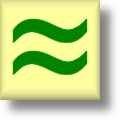Estimation Worksheets

## Dynamically Created Estimation Worksheets

Here is a graphic preview for all of the estimation worksheets. You can select different variables to customize these estimation worksheets for your needs. The estimation worksheets are randomly created and will never repeat so you have an endless supply of quality estimation worksheets to use in the classroom or at home. Our estimation worksheets are free to download, easy to use, and very flexible.

## Quick Link for All Estimation Worksheets

Click the image to be taken to that Estimation Worksheet.

##### Estimation WorksheetsSums and/or Differences2 Digits with Rounding Guide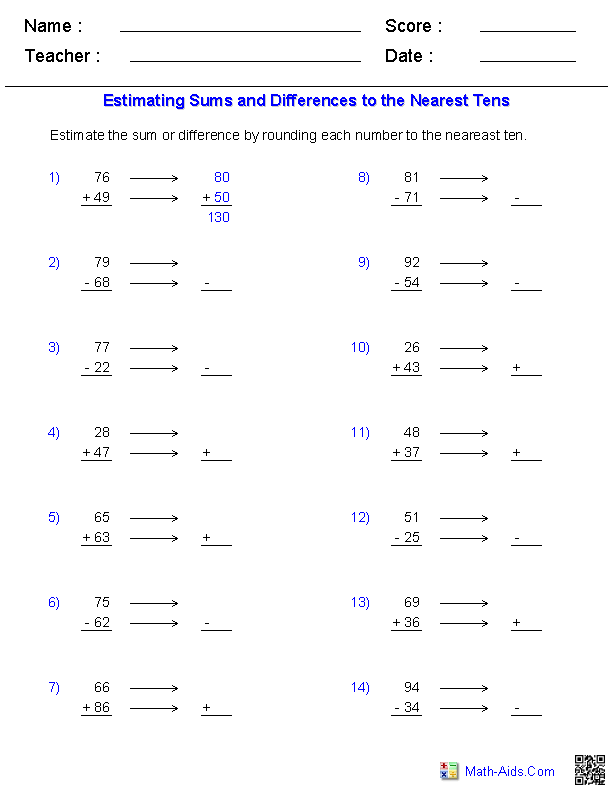##### Estimation WorksheetsSums and/or Differences3 Digits with Rounding Guide##### Estimation WorksheetsSums and/or Differences4 Digits with Rounding Guide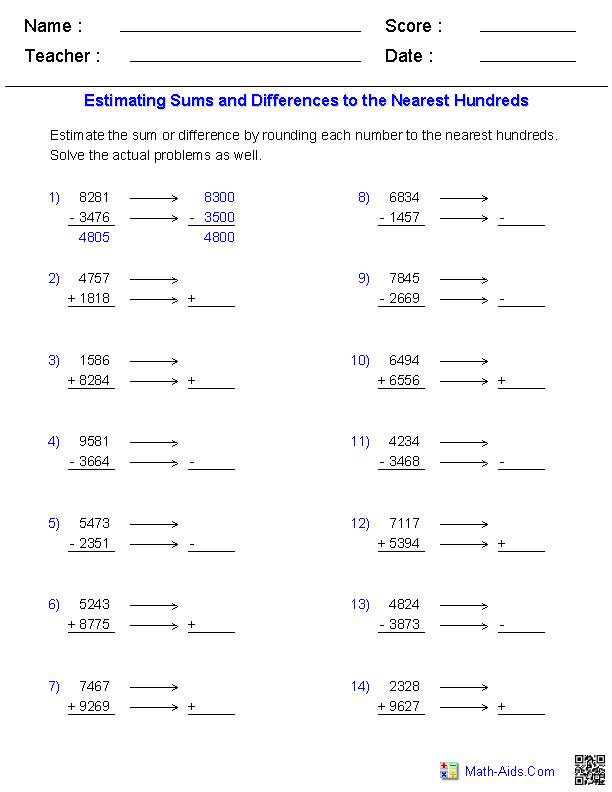##### Estimation WorksheetsProducts - 2 Digitswith Rounding Guide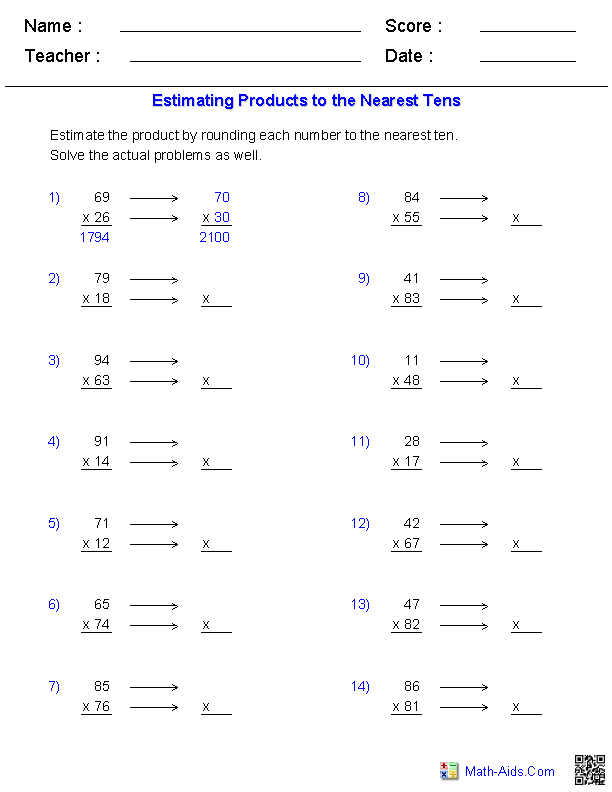##### Estimation WorksheetsProducts - 2 & 3 Digitswith Rounding Guide##### Estimation WorksheetsProducts - 3 Digitswith Rounding Guide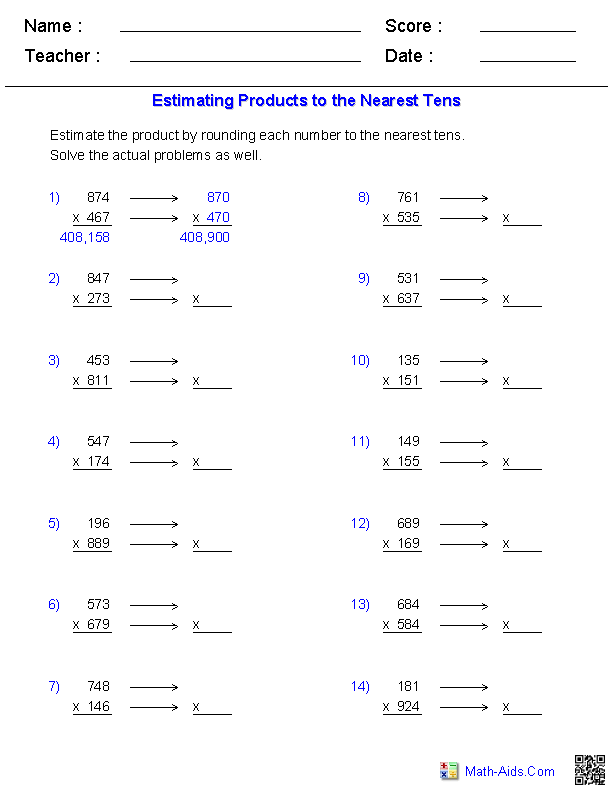##### Estimation WorksheetsSums and/or Differences2 Digits Horizontal Format##### Estimation WorksheetsSums and/or Differences3 Digits Horizontal Format##### Estimation WorksheetsSums and/or Differences4 Digits Horizontal Format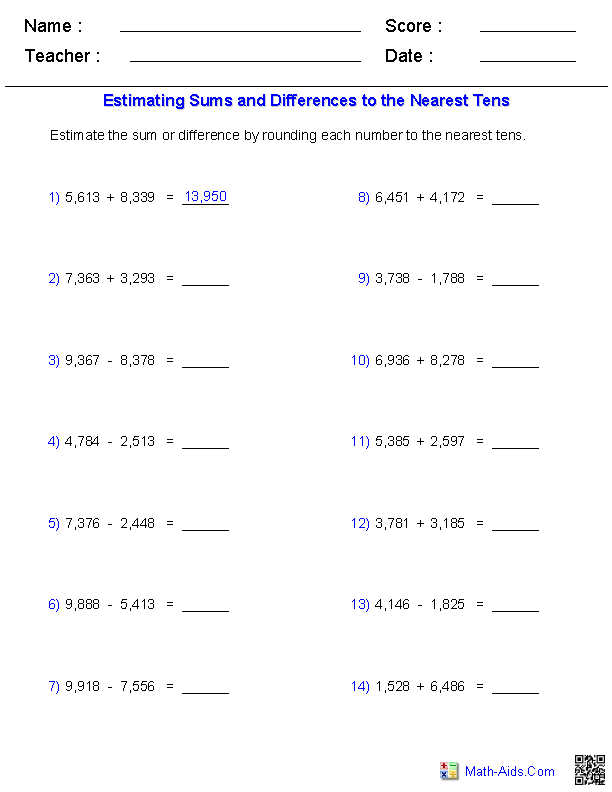##### Estimation WorksheetsProducts - 2 DigitsHorizontal Format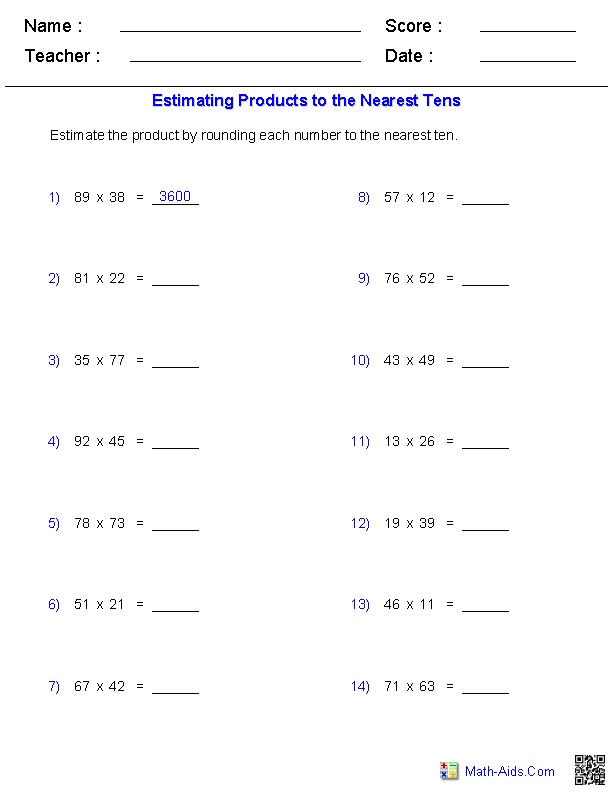##### Estimation WorksheetsProducts - 2 & 3 DigitsHorizontal Format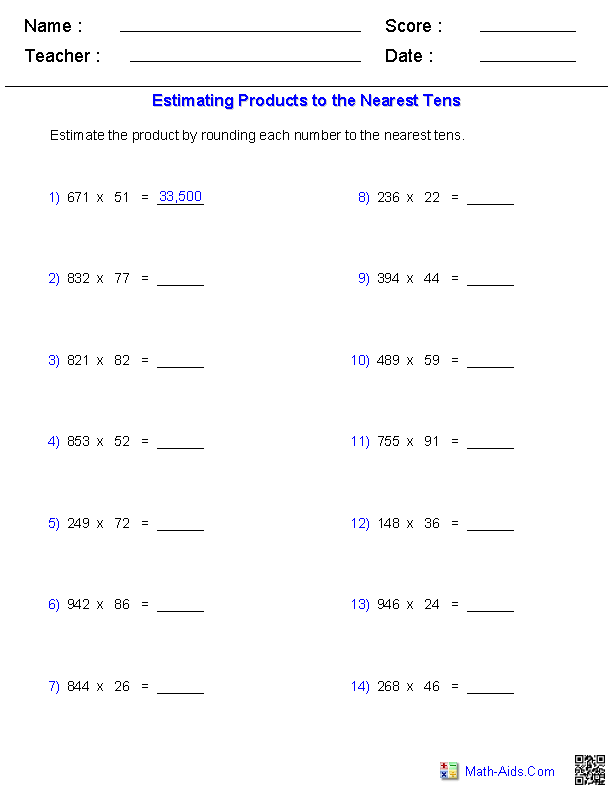##### Estimation WorksheetsProducts - 3 DigitsHorizontal Format##### Estimation WorksheetsSums and/or Differences2 Digits Word Problems##### Estimation WorksheetsSums and/or Differences3 Digits Word Problems##### Estimation WorksheetsSums and/or Differences4 Digits Word Problems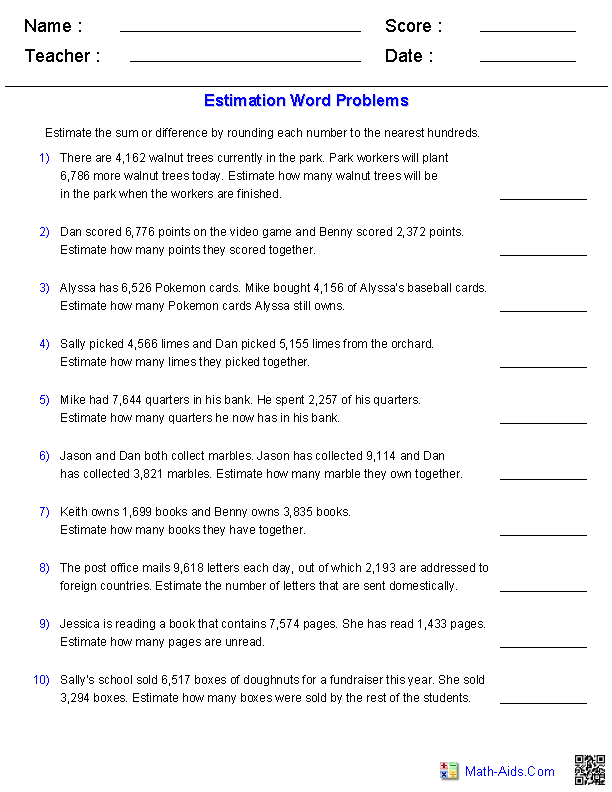Recommended Videos

## Detailed Description for All Estimation Worksheets

Sums and/or Differences Worksheets - 2 Digits with Rounding Guide
This estimation worksheet will produce 2 digit sums and differences problems with rounding guides for the students to solve. The students will round and rewrite the numbers in the place holder to the right of each problem. You may also instruct the students to solve the actual problems so they can compare the actual to the estimated solutions. You may select to have the first problem worked out as an example.

Sums and/or Differences Worksheets - 3 Digits with Rounding Guide
This estimation worksheet will produce 3 digit sums and differences problems with rounding guides for the students to solve. The students will round and rewrite the numbers in the place holder to the right of each problem. You may select either tens or hundreds for the students to round to for the problems. You may also instruct the students to solve the actual problems so they can compare the actual to the estimated solutions. You may elect to have the first problem worked out as an example.

Sums and/or Differences Worksheets - 4 Digits with Rounding Guide
This estimation worksheet will produce 4 digit sums and differences problems with rounding guides for the students to solve. The students will round and rewrite the numbers in the place holder to the right of each problem. You may select either tens, hundreds, or thousands for the students to round to for the problems. You may also instruct the students to solve the actual problems so they can compare the actual to the estimated solutions. You may elect to have the first problem worked out as an example.

Products Worksheets - 2 Digits Worksheets with Rounding Guide
This estimation worksheet will produce 2 digit multiplication problems with rounding guides for the students to solve the products. The students will round and rewrite the numbers in the place holder to the right of each problem. You may also instruct the students to solve the actual problems so they can compare the actual to the estimated solutions. You may select to have the first problem worked out as an example.

Products Worksheets - 2 and 3 Digits with Rounding Guide
This estimation worksheet will produce 2 and 3 digit multiplication problems with rounding guides for the students to solve the products. The students will round and rewrite the numbers in the place holder to the right of each problem. You may also instruct the students to solve the actual problems so they can compare the actual to the estimated solutions. You may select to have the first problem worked out as an example.

Products Worksheets - 3 Digits with Rounding Guide
This estimation worksheet will produce 3 digit multiplication problems with rounding guides for the students to solve the products. The students will round and rewrite the numbers in the place holder to the right of each problem. You may also instruct the students to solve the actual problems so they can compare the actual to the estimated solutions. You may select to have the first problem worked out as an example.

Sum and Differences Worksheets - 2 Digits Horizontal Format
This estimation worksheet will produce 2 digit sums and differences problems in a horizontal format for the students to solve. You may select to have the first problem worked out as an example.

Sum and Differences Worksheets - 3 Digits Horizontal Format
This estimation worksheet will produce 3 digit sums and differences problems in a horizontal format for the students to solve. You may select either tens or hundreds for the students to round to for the problems. You may elect to have the first problem worked out as an example.

Sum and Differences Worksheets - 4 Digits Horizontal Format
This estimation worksheet will produce 4 digit sums and differences problems in a horizontal format for the students to solve. You may select either tens, hundreds, or thousands for the students to round to for the problems. You may elect to have the first problem worked out as an example.

Products Worksheets - 2 Digits Horizontal Format
This estimation worksheet will produce 2 digit multiplication problems in a horizontal format for the students to solve the products. You may select to have the first problem worked out as an example.

Products Worksheets - 2 and 3 Digits Horizontal Format
This estimation worksheet will produce 2 and 3 digit multiplication problems in a horizontal format for the students to solve the products. You may select to have the first problem worked out as an example.

Products Worksheets - 3 Digits Horizontal Format
This estimation worksheet will produce 3 digit multiplication problems with rounding guides for the students to solve the products. The students will round and rewrite the numbers in the place holder to the right of each problem. You may also instruct the students to solve the actual problems so they can compare the actual to the estimated solutions. You may select to have the first problem worked out as an example.

Sum and Differences Word Problems - 2 Digits
This estimation worksheet will produce 2 digit sums and/or differences word problems for the students to solve. You can select sum, difference or a mixture of both types of problems.

Sum and Differences Word Problems - 3 Digits
This estimation worksheet will produce 3 digit sums and/or differences word problems for the students to solve. You can select sum, difference or a mixture of both types of problems.

Sum and Differences Word Problems - 4 Digits
This estimation worksheet will produce 4 digit sums and/or differences word problems for the students to solve. You can select sum, difference or a mixture of both types of problems.